## computer programsJOURNAL OFSYNCHROTRONRADIATION
ISSN: 1600-5775
Volume 28| Part 4| July 2021| Pages 1267-1272

aRIKEN SPring-8 Center, Koto 1-1-1, Sayo, Hyogo 679-5148, Japan
*Correspondence e-mail: ztanaka@spring8.or.jp

(Received 7 March 2021; accepted 17 April 2021; online 11 June 2021)

Since the first release in 2001, the synchrotron radiation calculation code SPECTRA has been used by many users and continuously upgraded to answer the requests and feedbacks from the users. Although such an incremental upgrade made in nearly two decades has significantly enhanced the capability of SPECTRA, the source code has become much more complicated, and thus the maintenance and further improvement are rather troublesome. To solve this issue, and to take advantage of the recent progress in the programming language, a major upgrade has been made as presented in this paper, in which a number of new features and advanced functions are implemented as well.

### 1. Introduction

SPECTRA is a computer program to numerically calculate the characteristics of synchrotron radiation (SR) emitted from various SR sources. Since the first release in 2001 (Tanaka & Kitamura, 2001), SPECTRA has been used by many users working in the field of SR science, and continuously upgraded to improve its features and add new functions (Tanaka & Kitamura, 2007; Tanaka, 2017a) to answer the requests and feedback from the users. Because of such an incremental upgrade made over nearly two decades, the source code has become much more complicated than what was first released, and its maintenance (bug fix) and improvement (adding new functions) are rather troublesome. In addition, the input (parameter) file of SPECTRA is written with an original syntax and is not necessarily easy to read, which makes it difficult to communicate with other external codes for the purpose of performing the so-called start-to-end simulations.

Another problem in the current version of SPECTRA is how to visualize the calculation results (output data), whose data structure is multidimensional (larger than 2); in the early versions, the maximum dimension (number of independent variables) of the output data was 2, and thus its visualization was rather simple. This is not the case for the recent versions, which potentially generate multidimensional data, such as the Wigner functions as described later.

Besides the above issues, we should turn to the rapid progress of programming languages. For example, the C++ language, which is chosen for the numerical code of SPECTRA, has been greatly revised and many useful functions have been added. In particular, C++11, a major revision made in 2011, where many improvements have been made not only in new features but also in grammatical descriptions that are useful for programmers of numerical codes. In addition, we have now a large number of cross-platform languages to write a code for graphical user interfaces (GUIs).

Considering the situations described above, we decided to upgrade SPECTRA on a major scale, in which many improvements are made to facilitate its operation, including a support to communicate with other codes. The purpose of this paper is to make an announcement of the major upgrade of SPECTRA, not only to introduce SPECTRA to potential users but also to inform the existing users who are familiar with the current version about important revisions made in the upgrade.

### 2. Overview of the program

Before reporting on the details of the major upgrade, an overview of SPECTRA is given first. The new version, as in the former ones, is composed of two parts: GUI and solver. The GUI helps the user to configure the parameters regarding the electron beam, light source, observation conditions and options for numerical operations (hereafter simply referred to as configurations) necessary to start a calculation, and generates an input file to be used in the solver. The solver performs numerical calculations with the configurations loaded from the input file. Note that the GUI invokes the solver as a child process, and receives the calculation progress from the solver, which is indicated in the GUI window to let the user know about the calculation status. Upon completion of the calculation, an output file is generated by the solver and is loaded in the GUI for visualization.

In the new version, the GUI is based on web technologies: HTML (HyperText Markup Language), CSS (Cascading Style Sheets) and Javascript. Although they have been originally developed to make the web site more convenient and useful, they can be nowadays applied to build a standalone desktop application that runs on a local computer, with the assistance of Node.js (Javascript runtime environment to run Javascript code without a web browser; https://nodejs.org/en/), Electron (framework to build a desktop application with Javascript, HTML and CSS; https://www.electronjs.org/) and Electron Builder (packaging manager for applications built with Electron; https://www.electron.build/). Among possible solutions to build the SPECTRA GUI, this option has been chosen in the new version because of a number of reasons, such as the portability between different platforms and many useful libraries available freely online.

The solver is written in the C++ language (C++11), and is built with several header-only libraries to facilitate numerical operations. Besides the normal usage (being invoked from the GUI), it can be independently run as a standalone program, using an input file prepared separately by the user. Note that parallel computing based on the Message Passing Interface (MPI) is supported to reduce the computation time. Although running the solver independently of the GUI was possible even in the former versions, it was not officially supported.

Unlike other computer programs to calculate the characteristics of SR, such as SRW (Chubar & Elleaume, 1998) and XOP (del Rio & Dejus, 2011) that are equipped with functions to design the SR beamline through the ray-tracing and wavefront-propagation methods, SPECTRA is dedicated to the characterization and modeling of SR sources with a wide variety of options. It should be emphasized, however, that SPECTRA has recently implemented an option to enable the wavefront propagation by means of the coherent mode decomposition, which will be described in detail later.

### 3. New features

Now let us explain the new features implemented in the major upgrade.

#### 3.1. Input and output formats

Defining the format of the input file is an important task to develop a computer program to deal with a large number of parameters, which is also the case for SPECTRA. In the former versions of SPECTRA, the input format was defined according to an original syntax and was rather inefficient, which made the input file difficult to read. To improve the readability of the input file of SPECTRA, we decided to change its format in the new version. Among a number of possible solutions, we have chosen the JavaScript Object Notation (JSON) format because of many advantages such as the simple syntax and structure, compatibility to many programming languages (besides Javascript), and capability to hold many data types (number, array and character string).

In addition to revising the format of the input file as described above, that of the output file has been modified as well. In the former versions, a simple text (ASCII) file was generated as an output file to save the calculation result as tabulated data with a header line to identify each column. Although such a format is simple and easy to read, we have had three major problems, as follows. First, no information about the calculation configurations is recorded. Second, loading the multidimensional data for visualization is complicated. Third, the file size can be much larger than what is really needed, especially for multidimensional data. To overcome these difficulties, the JSON format has been chosen as the new format of the output file. Note that the calculation conditions, as well as the calculation result, are recorded in the output file in the new version, and can be loaded in the GUI. This makes it possible to verify the condition assumed in a specific output file, or repeat similar calculations with different configurations.

As described above, the input and output files of the new version are not compatible with those of the former versions. To avoid potential problems coming from the incompatibility, SPECTRA offers two solutions. First, a utility program is available to convert existing input files written in the former format to those in the new format. Second, generating an output file in the former ASCII format is optionally possible.

#### 3.2. Multidimensional data

In the early versions of SPECTRA, the number of independent variables in a calculation was at most 2, and thus defining the format of the output file was rather simple. In the recent versions, however, there exist several cases where the number of variables is larger than 2, and the output data are multidimensional. The Wigner function, first introduced in quantum mechanics (Wigner, 1932) and later applied to represent the photon density in the four-dimensional (4D) phase space (x, y, x′, y′) (Coisson & Walker, 1986; Kim, 1987), is an example. The volume power density, which has been first implemented in the new version and will be explained later in detail, is also represented by multidimensional data.

Another case, which potentially generates the multidimensional data, is to make use of an option to scan a parameter; to investigate the effects due to variation of a specific parameter, SPECTRA offers an option to repeat calculations with the parameter being scanned in a given range. In the former versions, calculation results with this option are saved separately in different output files. To facilitate visualization of the scanning results, the new version has a function to bundle all the results into a single data set, and save it in a single output file. In such a case, the output data can be multidimensional.

#### 3.3. Post-processing

In the former versions of SPECTRA, a simple graphical plotter was available to post-process (visualize) the calculation results. Although it was useful to quickly check the calculation results, its function was rather limited. In addition, visualization of the multidimensional data as described in the previous section was not available. In the new version, we significantly enhanced the function of the post-processor, with the assistance of Plotly (JavaScript open source graphing library; https://plotly.com/javascript/).

One example of the new post-processor to visualize the multidimensional data is shown in Fig. 1, in which a Wigner function at the photon energy of 300 eV calculated in the 4D phase space with the parameters listed in Appendix A(Light source 1 in Table 1) is visualized. Because plotting the 4D data as a single graph is generally impossible, 2D data sliced at (x, x′) = (0, 0) are plotted in the (y, y′) phase space as a surface plot in this example. Note that the slicing position can be arbitrarily chosen by the user.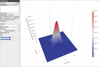Figure 1 Example of the new post-processor: visualization of the 4D Wigner function.

Another example is to view the scanning results as an animation. To demonstrate this function, a series of spectra of undulator radiation with different K values were calculated by means of the parameter scan' option, using the parameters listed in Appendix A(Light source 2). Then, an animation formed by a number of frames, each of which corresponds to one of the spectra, was generated and is provided in the supporting information. Figure 2shows a snapshot of the animation showing a spectrum with the fixed K value of 2, in which brilliance curves up to the ninth harmonics are plotted as well; the brilliance curve is defined as an envelope of maximum brilliance values at each harmonic, which are retrieved from the spectral profiles with different K values, and is often used to represent the performance of an undulator as a light source. Note that the brilliance in this example is evaluated under a Gaussian approximation, and thus a prefix GA.' is attached.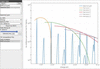Figure 2 Example of the new post-processor: snapshot of an animation to view the scanning results. The blue line shows the spectrum at the fixed K value of 2, while others show the brilliance curves up to the ninth harmonics.

#### 3.4. Solver in a standalone mode and Python support

Running the solver independently of the GUI is now officially supported. To do so, the user should prepare an input JSON file that contains all the configurations necessary to start a calculation with the solver. Although all of the configurations are explained in the reference manual together with the syntax, it is recommended to create a master' input file in the GUI, and modify a part of the configurations for another calculation. This helps to minimize the possibility of unexpected errors in the input file. Then, run the solver with the input file given as an argument. For details of the usage of the solver, refer to the reference manual. Upon completion, the solver generates an output file in the JSON format, which can be imported later in the post-processor in the GUI for visualization, as in the normal usage.

Besides the above method to run the solver, SPECTRA offers a Python extension library, so that a Python script can access the functions of the solver. Note that the library is written in the C++ language with most of the code common to the solver, and is built with pybind11 (header-only library to create Python bindings of existing C++ code; https://github.com/pybind/pybind11). Although an input file is still needed to create an instance of the solver' class, it provides a number of functions to modify the configurations, to start the calculation, and to retrieve the result as a Python native data type (list). This option offers a more flexible usage of the solver, such as optimization of a number of parameters, and communication with other codes.

#### 3.5. Web-application mode

Thanks to the GUI libraries based on the web technologies, the new version of SPECTRA runs as a web-application as well as the standalone desktop application. In this mode, the users do not have to install SPECTRA in their local computers; instead, they access a web server in which SPECTRA runs in a web-application mode. To be specific, SPECTRA installed in the server (which may probably be a high-performance computer) runs as an HTTP/HTTPS server, receives commands from the user through a web browser, invokes the solver (installed in the same server) as a child process, displays the calculation progress, and visualizes the calculation results in the post-processor. Because the output file is saved in the server but not in the local computer, the users may need to download it for their own applications.

Besides the new features of SPECTRA implemented in the major upgrade as presented in the previous section, there are several advanced functions implemented or improved in the new version. Among them, two functions are presented in this section.

#### 4.1. Volume power density

The volume power density is defined as the time-averaged radiation power absorbed per unit volume in an object illuminated by SR. This can be used in a numerical simulation based on the finite element method, to be performed to investigate the deformation of the beamline components such as beam shutters, mirrors and monochromators. In contrast to the spatial power density, or the radiation power passing through a unit area, the volume power density is calculated as a function of not only the transverse coordinate x and y but also the depth D, the distance from the surface of the object. In a mathematical form, it is defined aswhere d2F/dxdy denotes the photon flux density at the photon energy of ℏω, μ and μen are the linear attenuation and energy absorption coefficients, respectively, D is the distance from the surface of the object (`depth'), and C is a unit conversion factor. Note that μen describes the fraction of radiation energy absorbed by the object per unit depth, and should be distinguished from μ. In low-energy regions where the photoelectric absorption is the only effect to attenuate radiation, we have μenμ. This is not the case in high-energy regions where the Compton scattering and pair creation are dominant. In SPECTRA, μ is calculated with the assitance of mucal.c (C source code freely available online; http://www.csrri.iit.edu/mucal.html) and μen is evaluated by interpolating the values given in the NIST database (database for the mass energy-absorption coefficients; https://www.nist.gov/pml/X-ray-mass-attenuation-coefficients).

Besides the simple condition in which SR is normally incident on a target object, SPECTRA supports glancing-incidence conditions; an example is schematically illustrated in Fig. 3, where SR emitted from the source is first collimated by a slit to confine the angular acceptance, and is incident on the inclined target object. One of the typical components supposed in this example is a vertically dispersive optical element; note that horizontally dispersive elements are also available by specifying the direction (azimuth) of incidence.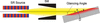Figure 3 Schematic illustration of glancing incidence: vertically dispersive elements are assumed in this example.

Figure 4shows an example of the volume power density sliced at x = 0 and plotted in the (y, D) plane. The calculation was performed using the parameters listed in Appendix A(Light source 2, with the K value of 1 corresponding to the fundamental photon energy of 12.7 keV), assuming that a target object made of carbon is located 30 m far from the light source and is tilted by 30° (= the incidence angle). The angular acceptance is assumed to be 0.06 mrad.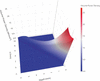Figure 4 Example of the volume power density calculation under a glancing-incidence condition.

#### 4.2. Facilitating the coherent mode decomposition

The coherent mode decomposition (CMD) is one of the mathematical methods to describe the propagation of partially coherent radiation. In the CMD method, the radiation is decomposed into a number of coherent modes whose propagation can be fully described by wave optics. The conventional CMD method is based on the decomposition of cross spectral density, which reduces to solving a matrix eigenvalue problem, and may require a high numerical cost in terms of the computation time and required memory.

Recently, we developed a numerical method to perform the CMD using a Wigner function (Tanaka, 2017b), and implemented in the former version (10.1) of SPECTRA. In this method, we first calculate the Wigner function W in the 4D phase space with a reasonable range and number of points. Then, we assume that the complex amplitude of the pth coherent mode Ψp is given aswithbeing the mth-order Hermite–Gaussian (HG) function, where wx and wy are the beam waist parameters to normalize the transverse coordinate, ah,j is the amplitude of the HG function of ψhψj, Hm is the Hermite polynomial of the order m, and Np is the maximum order of the HG modes to form the pth coherent mode. Note that the indices h and j are given as a function of the integer q and order p.

To determine the coefficients ah,j, we consider a function W′ defined aswheredenotes an operator to form a Wigner function from the complex amplitude Ψp, and Mc is the maximum order of the coherent modes. In SPECTRA, ah,j is numerically determined so that the reconstructed Wigner function W′ reproduces the original function W. Once they are determined, the complex amplitude of each coherent mode can be evaluated using equation (2) to describe its propagation through the optical system in the SR beamline. Note that the unit of ah,j can be arbitrarily chosen, as long as the operatorgenerates W′ with the same unit as W. In SPECTRA, ah,j is dimensionless and defined to satisfy a conditionif the CMD is perfect.

Although the CMD method implemented in SPECTRA was numerically cost effective and could be done with an ordinary computer, trial-and-error processes might be needed to optimize wx, wy and Np, to obtain a reliable and reasonable result. This makes the CMD method rather difficult to use in the former version. To overcome this difficulty, we implemented an automatic optimizer for these parameters and used in the new version. As a result, the CMD can be performed without any trial-and-error processes, in a much simpler manner than the former versions.

As an example, the CMD method in the new version was demonstrated using the 4D Wigner function whose sliced profile is shown in Fig. 1. Using the coefficients ah,j determined in the CMD process, the spatial profiles of complex amplitude Ψp (real part) were calculated and plotted in Fig. 5, for four different values of the order of the coherent mode (p = 0, 1, 5 and 10). It is worth noting that a higher order results in a broader profile only in the horizontal direction, which is attributable to the coherence in the horizontal direction being much worse than in the vertical. More details about how Ψp varies as p (from 0 to 100) can be seen as an animation in the supporting information.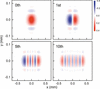Figure 5 Spatial profiles of Ψp, the complex amplitude of the pth coherent mode, for different values of p.

To perform the wavefront propagation using the coherent modes described above, how to specify the maximum order Mc is an important issue. One criterion is the integrated modal fluxdefined aswhich means the percentage of photon flux contained in the coherent modes up to the Mcth order. Because ah,j is defined to satisfy the normalization condition (5),approaches unity for larger Mc values as shown in Fig. 6.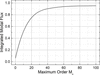Figure 6 Integrated modal fluxas a function of the maximum order of the coherent modes Mc.

It is easy to specify the reasonable value of Mc from this figure. As an example, let≃ 0.95 be a criterion, meaning that 95% of the flux is contained in the coherent modes. Then, we have a reasonable value of Mc = 80 in this example.

### 5. Summary

We reported on the major upgrade of SPECTRA. Note that the latest version of SPECTRA as of March 2021 is 11.0, and it is freely available online (https://spectrax.org/spectra/). We hope that the new features and advanced functions implemented in this version contribute to the advancement of SR science.

### Parameters used in the calculations

Electron beam and light source parameters used in the example calculations, whose results are shown in Figs. 1, 2, 4, 5and 6, are listed in Table 1. Note that two light sources for soft and hard X-ray regions are considered.

 Table 1Electron beam and light source parameters
 Electron beam Energy 8 GeV Average current 100 mA Natural emittance 2.4 nm rad Coupling constant 0.002 Energy spread 1.1 × 10−3 Horizontal betatron function 31.2 m Vertical betatron function 5 m Dispersion function 0.146 m Light source 1 Type Helical undulator Period 120 mm Total length 1.5 m K value 4.39 Fundamental energy 300 eV Light source 2 Type Linear undulator Period 32 mm Total length 4.5 m Maximum K value 2.5 Fundamental energy for K = 1 12.7 keV

### Acknowledgements

The author thanks Dr H. Ohashi of Japan Synchrotron Radiation Research Institute for discussions on the volume power density.

### ReferencesJOURNAL OFSYNCHROTRONRADIATION